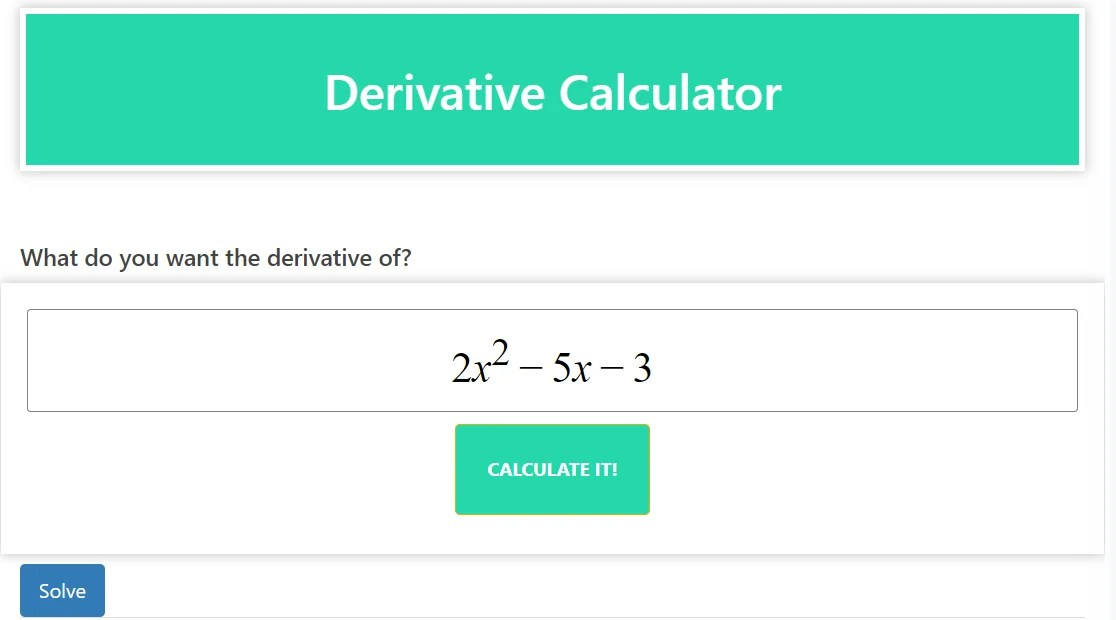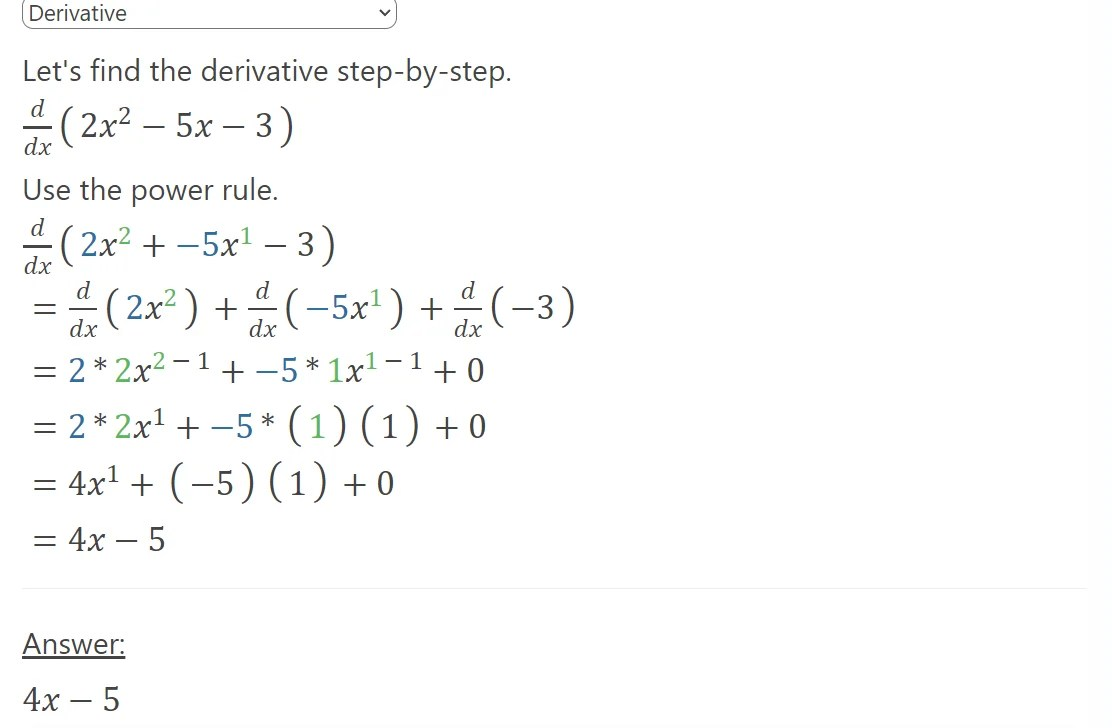# Derivative Calculator (Maths solver)

A derivative calculator, also referred to as a math solver or a differentiation calculator, is a tool or software program specifically designed to compute the derivative of a given mathematical function. In the realm of mathematics, the derivative signifies the rate at which a function changes concerning its independent variable.

The primary purpose of a derivative calculator is to automate the process of finding derivatives. Calculating derivatives by hand can often be complex and time-consuming, particularly for more intricate functions. This is where a derivative calculator proves to be immensely useful. It eliminates the need for manual calculations and allows users to obtain quick and accurate results.

### The functionality of a derivative calculator

The functionality of a derivative calculator may vary depending on the specific tool or software program being used. However, most derivative calculators are equipped to handle a wide range of functions and mathematical operations. They can process basic functions such as polynomials, trigonometric functions (like sine, cosine, tangent, etc.), exponential functions, logarithmic functions, and more.

Derivative calculators employ algorithms and mathematical rules to compute derivatives. These algorithms incorporate various differentiation rules, such as the power rule, product rule, quotient rule, chain rule, and trigonometric identities. By applying these rules, the calculator simplifies and solves the given function, enabling it to compute the derivative accurately.

### Using a derivative calculator

Using a derivative calculator is often a straightforward process. Users typically input the mathematical function for which they want to find the derivative. The calculator then processes the input, applies the necessary rules and algorithms, and generates the derivative as output. In some cases, derivative calculators may provide additional information, such as graphs or plots of the function and its derivative, offering a visual representation of the mathematical concept.

Derivative calculators find extensive usage among students, teachers, researchers, and professionals who work with mathematical functions. They serve as powerful tools for verifying manual calculations, checking answers, exploring the properties of functions, and solving mathematical problems involving derivatives. By streamlining the process of finding derivatives, derivative calculators contribute to increased efficiency and accuracy in mathematical analysis and problem-solving tasks.

To summarize, a derivative calculator (maths solver) is a specialized tool or software program that automates the process of finding derivatives. It saves time and effort by performing complex calculations and applying differentiation rules to a given mathematical function. Whether you are a student or a professional, a derivative calculator can assist you in solving a wide range of problems involving derivatives, providing quick and accurate results.